5.5.2 Step 2: Delete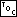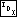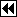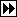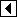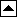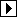At this point, we've identified p as the node to delete. The node on the top of the stack, da[k - 1], is p's parent node. There are the same three cases we saw in deletion from an ordinary BST (see Deleting from a BST), with the addition of code to copy balance factors and update the stack.

The code for selecting cases is the same as for BSTs:

```166. <Step 2: Delete item from AVL tree 166> =
if (p->avl_link == NULL)
{ <Case 1 in AVL deletion 168> }
else   {
struct avl_node *r = p->avl_link;
if (r->avl_link == NULL)
{         <Case 2 in AVL deletion 169>       }
else       {         <Case 3 in AVL deletion 170>       }
}
```

This code is included in 164.

Regardless of the case, we are in the same situation after the deletion: node p has been removed from the tree and the stack contains k nodes at which rebalancing may be necessary. Later code may change p to point elsewhere, so we free the node immediately. A pointer to the item data has already been saved in item (see avldelsaveitem):

```167. <Step 2: Delete item from AVL tree 166> +=
tree->avl_alloc->libavl_free (tree->avl_alloc, p);
```
##### Case 1: p has no right child

If p has no right child, then we can replace it with its left child, the same as for BSTs (see bstdelcase1).

```168. <Case 1 in AVL deletion 168> =
pa[k - 1]->avl_link[da[k - 1]] = p->avl_link;
```

This code is included in 166.

##### Case 2: p's right child has no left child

If p has a right child r, which in turn has no left child, then we replace p by r, attaching p's left child to r, as we would in an unbalanced BST (see bstdelcase2). In addition, r acquires p's balance factor, and r must be added to the stack of nodes above the deleted node.

```169. <Case 2 in AVL deletion 169> =
r->avl_balance = p->avl_balance;
pa[k - 1]->avl_link[da[k - 1]] = r;
da[k] = 1;
pa[k++] = r;
```

This code is included in 166.

##### Case 3: p's right child has a left child

If p's right child has a left child, then this is the third and most complicated case. On the other hand, as a modification from the third case in an ordinary BST deletion (see bstdelcase3), it is rather simple. We're deleting the inorder successor of p, so we push the nodes above it onto the stack. The only trickery is that we do not know in advance the node that will replace p, so we reserve a spot on the stack for it (da[j]) and fill it in later:

```170. <Case 3 in AVL deletion 170> =
struct avl_node *s;
int j = k++;

for (;;)   {
da[k] = 0;
pa[k++] = r;
if (s->avl_link == NULL)
break;

r = s;
}

s->avl_balance = p->avl_balance;

pa[j - 1]->avl_link[da[j - 1]] = s;
da[j] = 1;
pa[j] = s;
```

This code is included in 166.

Exercises:

1. Write an alternate version of <Case 3 in AVL deletion 170> that moves data instead of pointers, as in Exercise 4.8-2. [answer]

2. Why is it important that the item data was saved earlier? (Why couldn't we save it just before freeing the node?) [answer]